×# Unreal入门AO知识科普

Hello，大家好，今天是一篇Unreal入门AO知识科普分享，我是木偶心没。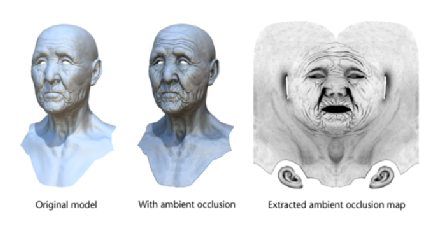AO，Ambient Occlusion，环境光遮蔽。主要是描述了表面上的任何一点所接受到的环境光被周围几何体所遮蔽的百分比，因此使得渲染的结果更加富有层次感，对比度更高。如下来自wiki的效果对比：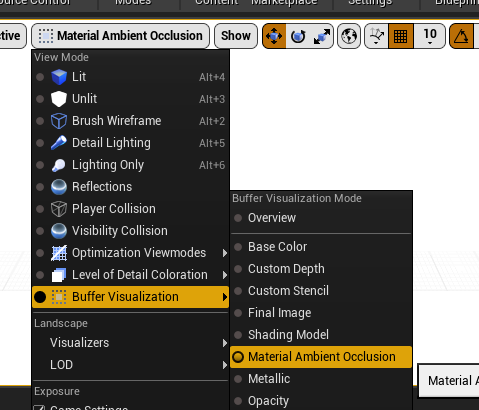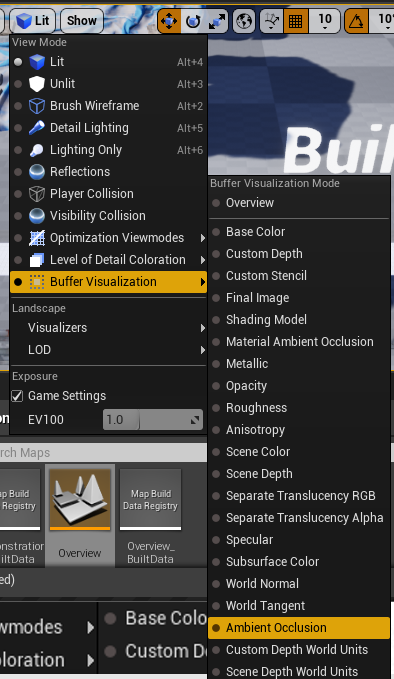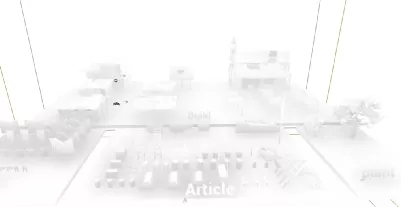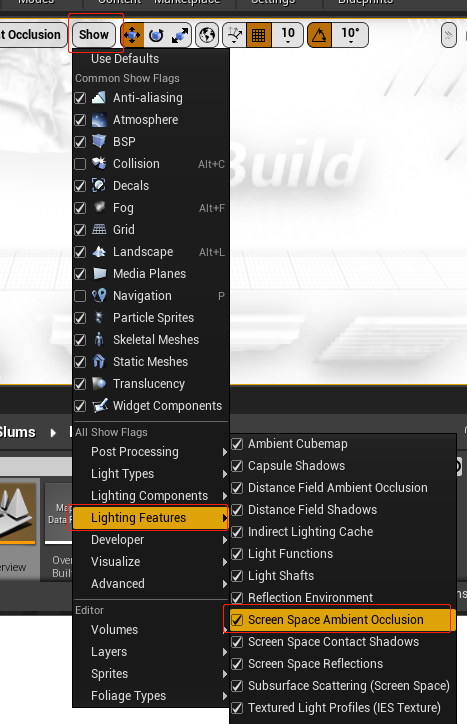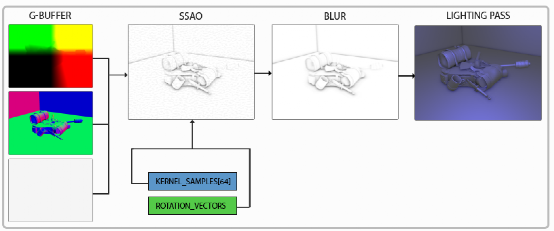SSAO是屏幕空间的AO，也就是不是场景的预处理，是屏幕后期处理，根据OpenGL的算法，要得到SSAO，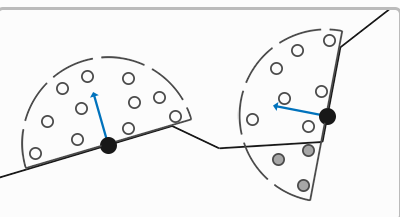1、计算AO

std::uniform_real_distributionrandomFloats(0.0, 1.0); // 随机浮点数，范围0.0 - 1.0

std::default_random_engine generator;

for (GLuint i = 0; i < 64; ++i)

{

glm::vec3 sample(

randomFloats(generator) * 2.0 - 1.0,

randomFloats(generator) * 2.0 - 1.0,

randomFloats(generator)

);

sample = glm::normalize(sample);

sample *= randomFloats(generator);

GLfloat scale = GLfloat(i) / 64.0;

ssaoKernel.push_back(sample);

}

2、模糊AO

3、混合

#version 330 core

out vec4 FragColor;

in vec2 TexCoords;

uniform sampler2D gPositionDepth;

uniform sampler2D gNormal;

uniform sampler2D gAlbedo;

uniform sampler2D ssao;

struct Light {

vec3 Position;

vec3 Color;

float Linear;

};

uniform Light light;

void main()

{

// 从G缓冲中提取数据

vec3 FragPos = texture(gPositionDepth, TexCoords).rgb;

vec3 Normal = texture(gNormal, TexCoords).rgb;

vec3 Diffuse = texture(gAlbedo, TexCoords).rgb;

float AmbientOcclusion = texture(ssao, TexCoords).r;

// Blinn-Phong (观察空间中)

vec3 ambient = vec3(0.3 * AmbientOcclusion); // 这里我们加上遮蔽因子

vec3 lighting  = ambient;

vec3 viewDir  = normalize(-FragPos); // Viewpos 为 (0.0.0)，在观察空间中

// 漫反射

vec3 lightDir = normalize(light.Position - FragPos);

vec3 diffuse = max(dot(Normal, lightDir), 0.0) * Diffuse * light.Color;

// 镜面

vec3 halfwayDir = normalize(lightDir + viewDir);

float spec = pow(max(dot(Normal, halfwayDir), 0.0), 8.0);

vec3 specular = light.Color * spec;

// 衰减

float dist = length(light.Position - FragPos);

float attenuation = 1.0 / (1.0 + light.Linear * dist + light.Quadratic * dist * dist);

diffuse  *= attenuation;

specular *= attenuation;

lighting += diffuse + specular;

FragColor = vec4(lighting, 1.0);

}1903学员阮捍、徐珂小组作品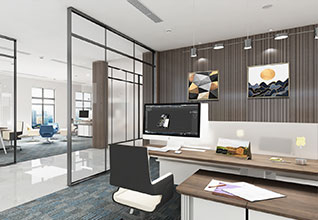1903学员周德志小组作品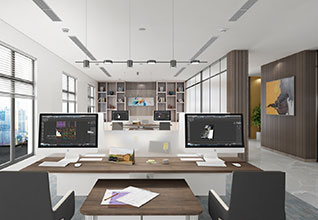1903学员周德志小组作品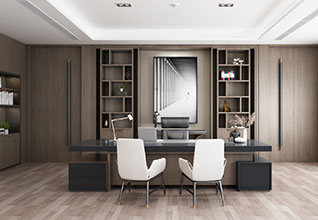1903学员王悦、王思琪小组作品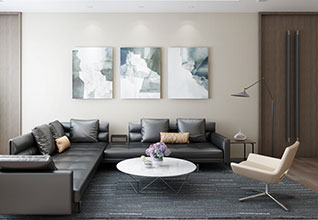1903学员王悦、王思琪小组作品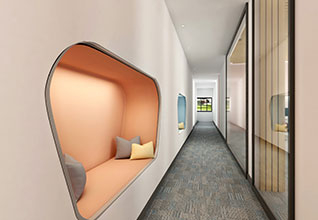1903期学员阮捍、徐珂小组作品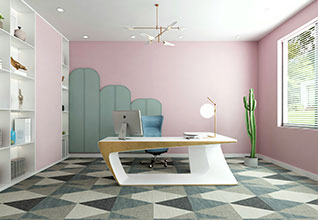1903期学员阮捍、徐珂小组作品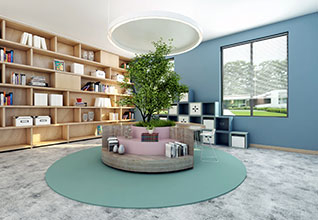1903期学员阮捍、徐珂小组作品